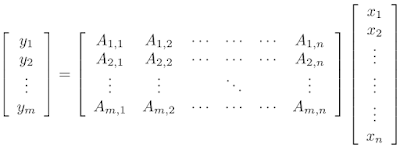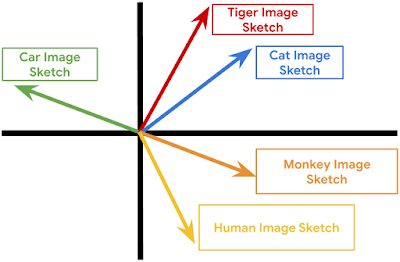# Recursive Sketches for Modular Deep Learning

Much of classical machine learning (ML) focuses on utilizing available data to make more accurate predictions. More recently, researchers have considered other important objectives, such as how to design algorithms to be small, efficient, and robust. With these goals in mind, a natural research objective is the design of a system on top of neural networks that efficiently stores information encoded within—in other words, a mechanism to compute a succinct summary (a “sketch”) of how a complex deep network processes its inputs. Sketching is a rich field of study that dates back to the foundational work of Alon, Matias, and Szegedy, which can enable neural networks to efficiently summarize information about their inputs.

For example: Imagine stepping into a room and briefly viewing the objects within. Modern machine learning is excellent at answering immediate questions, known at training time, about this scene: “Is there a cat? How big is said cat?” Now, suppose we view this room every day over the course of a year. People can reminisce about the times they saw the room: “How often did the room contain a cat? Was it usually morning or night when we saw the room?”. However, can one design systems that are also capable of efficiently answering such memory-based questions even if they are unknown at training time?

In “Recursive Sketches for Modular Deep Learning”, recently presented at ICML 2019, we explore how to succinctly summarize how a machine learning model understands its input. We do this by augmenting an existing (already trained) machine learning model with “sketches” of its computation, using them to efficiently answer memory-based questions—for example, image-to-image-similarity and summary statistics—despite the fact that they take up much less memory than storing the entire original computation.

Basic Sketching Algorithms
In general, sketching algorithms take a vector x and produce an output sketch vector that behaves like x but whose storage cost is much smaller. The fact that the storage cost is much smaller allows one to succinctly store information about the network, which is critical for efficiently answering memory-based questions. In the simplest case, a linear sketch x is given by the matrix-vector product Ax where A is a wide matrix, i.e., the number of columns is equal to the original dimension of x and the number of rows is equal to the new reduced dimension. Such methods have led to a variety of efficient algorithms for basic tasks on massive datasets, such as estimating fundamental statistics (e.g., histogram, quantiles and interquartile range), finding popular items (known as frequent elements), as well as estimating the number of distinct elements (known as support size) and the related tasks of norms and entropy estimation.A simple method to sketch the vector x is to multiply it by a wide matrix A to produce a lower-dimensional vector y.
This basic approach works well in the relatively simple case of linear regression, where it is possible to identify important data dimensions simply by the magnitude of weights (under the common assumption that they have uniform variance). However, many modern machine learning models are actually deep neural networks and are based on high-dimensional embeddings (such as Word2Vec, Image Embeddings, Glove, DeepWalk and BERT), which makes the task of summarizing the operation of the model on the input much more difficult. However, a large subset of these more complex networks are modular, allowing us to generate accurate sketches of their behavior, in spite of their complexity.

Neural Network Modularity
A modular deep network consists of several independent neural networks (modules) that only communicate via one’s output serving as another’s input. This concept has inspired several practical architectures, including Neural Modular Networks, Capsule Neural Networks and PathNet. It is also possible to split other canonical architectures to view them as modular networks and apply our approach. For example, convolutional neural networks (CNNs) are traditionally understood to behave in a modular fashion; they detect basic concepts and attributes in their lower layers and build up to detecting more complex objects in their higher layers. In this view, the convolution kernels correspond to modules. A cartoon depiction of a modular network is given below.This is a cartoon depiction of a modular network for image processing. Data flows from the bottom of the figure to the top through the modules represented with blue boxes. Note that modules in the lower layers correspond to basic objects, such as edges in an image, while modules in upper layers correspond to more complex objects, like humans or cats. Also notice that in this imaginary modular network, the output of the face module is generic enough to be used by both the human and cat modules.
Sketch Requirements
To optimize our approach for these modular networks, we identified several desired properties that a network sketch should satisfy:
• Sketch-to-Sketch Similarity: The sketches of two unrelated network operations (either in terms of the present modules or in terms of the attribute vectors) should be very different; on the other hand, the sketches of two similar network operations should be very close.
• Attribute Recovery: The attribute vector, e.g., the activations of any node of the graph can be approximately recovered from the top-level sketch.
• Summary Statistics: If there are multiple similar objects, we can recover summary statistics about them. For example, if an image has multiple cats, we can count how many there are. Note that we want to do this without knowing the questions ahead of time.
• Graceful Erasure: Erasing a suffix of the top-level sketch maintains the above properties (but would smoothly increase the error).
• Network Recovery: Given sufficiently many (input, sketch) pairs, the wiring of the edges of the network as well as the sketch function can be approximately recovered.This is a 2D cartoon depiction of the sketch-to-sketch similarity property. Each vector represents a sketch and related sketches are more likely to cluster together.
The Sketching Mechanism
The sketching mechanism we propose can be applied to a pre-trained modular network. It produces a single top-level sketch summarizing the operation of this network, simultaneously satisfying all of the desired properties above. To understand how it does this, it helps to first consider a one-layer network. In this case, we ensure that all the information pertaining to a specific node is “packed” into two separate subspaces, one corresponding to the node itself and one corresponding to its associated module. Using suitable projections, the first subspace lets us recover the attributes of the node whereas the second subspace facilitates quick estimates of summary statistics. Both subspaces help enforce the aforementioned sketch-to-sketch similarity property. We demonstrate that these properties hold if all the involved subspaces are chosen independently at random.

Of course, extra care has to be taken when extending this idea to networks with more than one layer—which leads to our recursive sketching mechanism. Due to their recursive nature, these sketches can be “unrolled” to identify sub-components, capturing even complicated network structures. Finally, we utilize a dictionary learning algorithm tailored to our setup to prove that the random subspaces making up the sketching mechanism together with the network architecture can be recovered from a sufficiently large number of (input, sketch) pairs.

Future Directions
The question of succinctly summarizing the operation of a network seems to be closely related to that of model interpretability. It would be interesting to investigate whether ideas from the sketching literature can be applied to this domain. Our sketches could also be organized in a repository to implicitly form a “knowledge graph”, allowing patterns to be identified and quickly retrieved. Moreover, our sketching mechanism allows for seamlessly adding new modules to the sketch repository—it would be interesting to explore whether this feature can have applications to architecture search and evolving network topologies. Finally, our sketches can be viewed as a way of organizing previously encountered information in memory, e.g., images that share the same modules or attributes would share subcomponents of their sketches. This, on a very high level, is similar to the way humans use prior knowledge to recognize objects and generalize to unencountered situations.

Acknowledgements
This work was the joint effort of Badih Ghazi, Rina Panigrahy and Joshua R. Wang.